# Basis maths-linear algebra-Q20

+1 vote

Let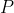be a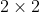matrix for which there is a constant k such that the sum of entries in each row and each column is k. Which of the following must be an eigenvector of A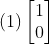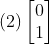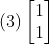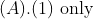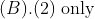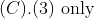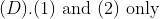asked May 15
reshown May 16answered Jun 11 by (112,760 points)